The restful feeling of happiness which is derived from the contemplation of the beauties of nature is one of the choicest blessings of existence; and in woodland scenery there is a mysterious charm that from the remotest ages has impressed itself upon the imagination of mankind.

Joseph Rodgers - The Scenery of Sherwood Forest, 1908.

I hope you find something that you like on this photo-blog.  These images show things that stop me, make me look, admire, connect, wonder and to sometimes just simply question what is or what might be going on.

Please also keep an eye on Adam Dunning's blog and also the galleries on his website.  Recent work includes his 'Beside the Seaside, Beside the Sea' gallery which has received very favourable reviews and his very latest gallery where he has captured images of 'Mr Marvels Showtime USA Fun Park'.  Adam's blog posts also feature some wonderful images from around Scarborough, Whitby, Filey, Bempton and Cayton plus Sunk Island on the north bank of the River Humber.

Mick Fattorini's exhibition 'Scarborough 1963 - 1970' at the Woodend Gallery, Scarborough has now closed although I popped along a few times for a good look.  For 2020, Mick will be exhibiting his latest work very soon in Leicester.  See here his website and for more information about the exhibitions.

Sciryuda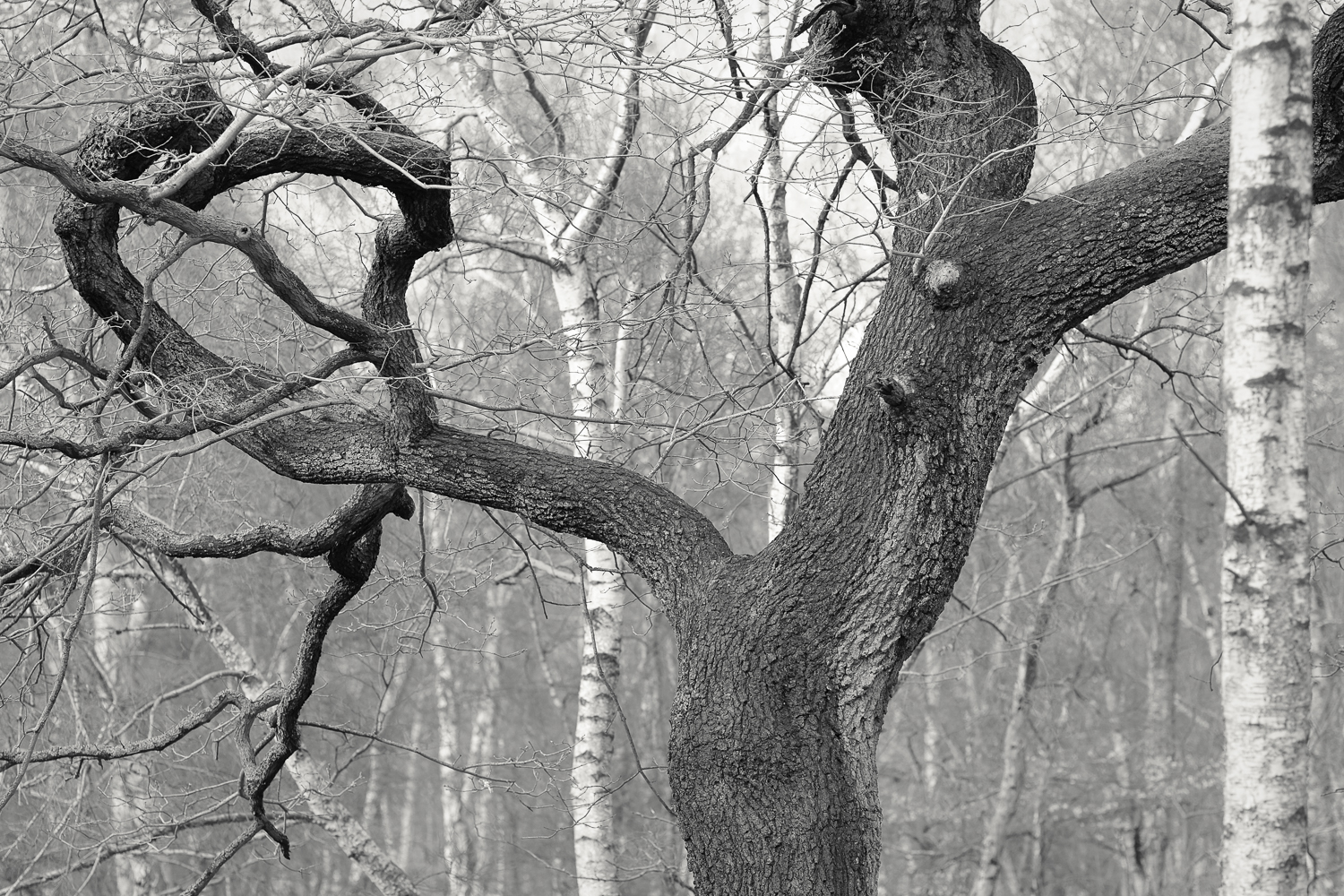Oak.  The Birklands

Sciryuda.  Sherwood Forest, Nottinghamshire.

SciryudaOak and Ash Field Markers.  West of the Lings.

Sciryuda.  Sherwood Forest, Nottinghamshire.

Sciryuda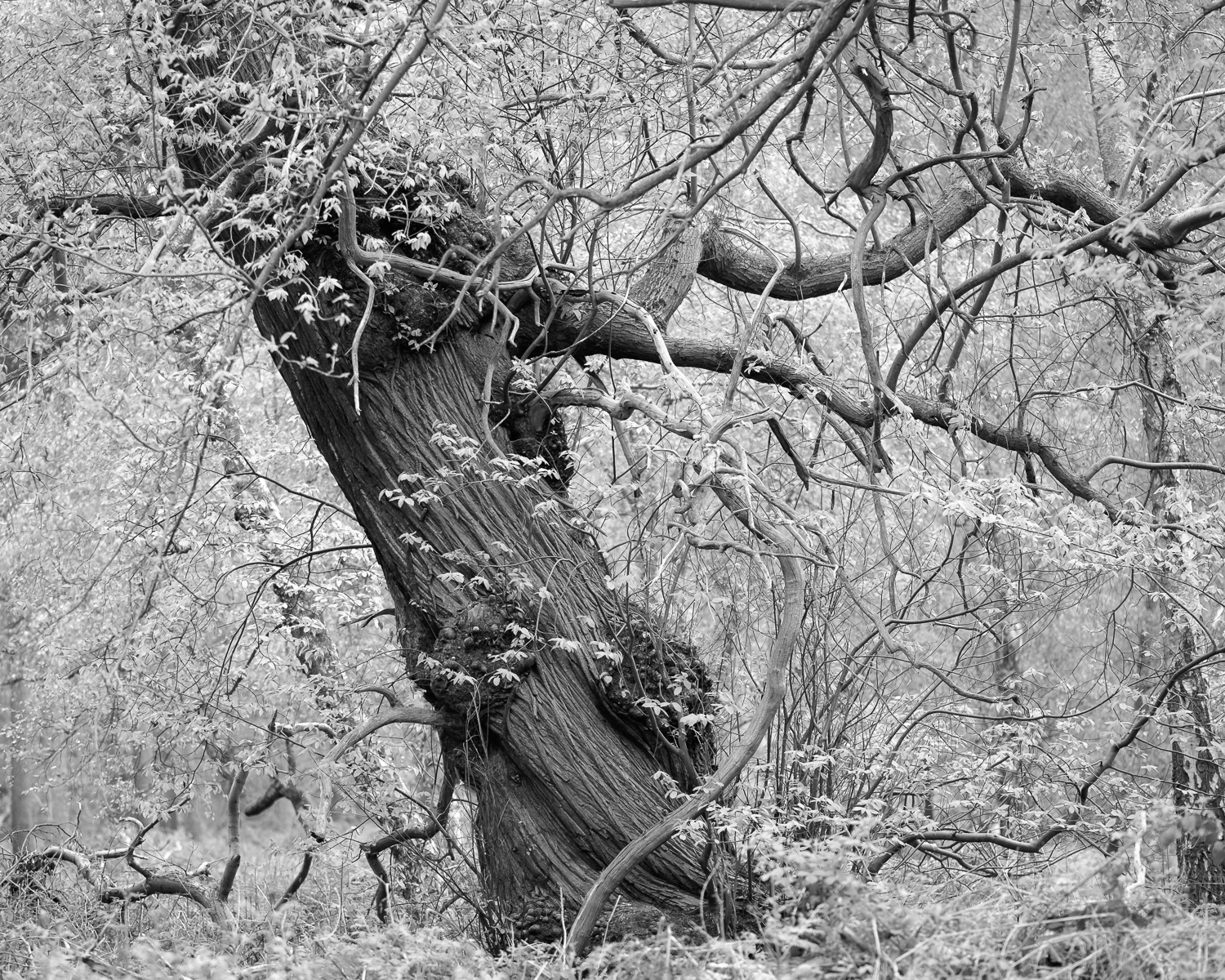Sweet Chestnut.  Drinking Pit Lane, Welbeck.

Sciryuda. Sherwood Forest, Nottinghamshire.

SciryudaOak.  The Birklands.

Sciryuda.  Sherwood Forest, Nottinghamshire.

Sciryuda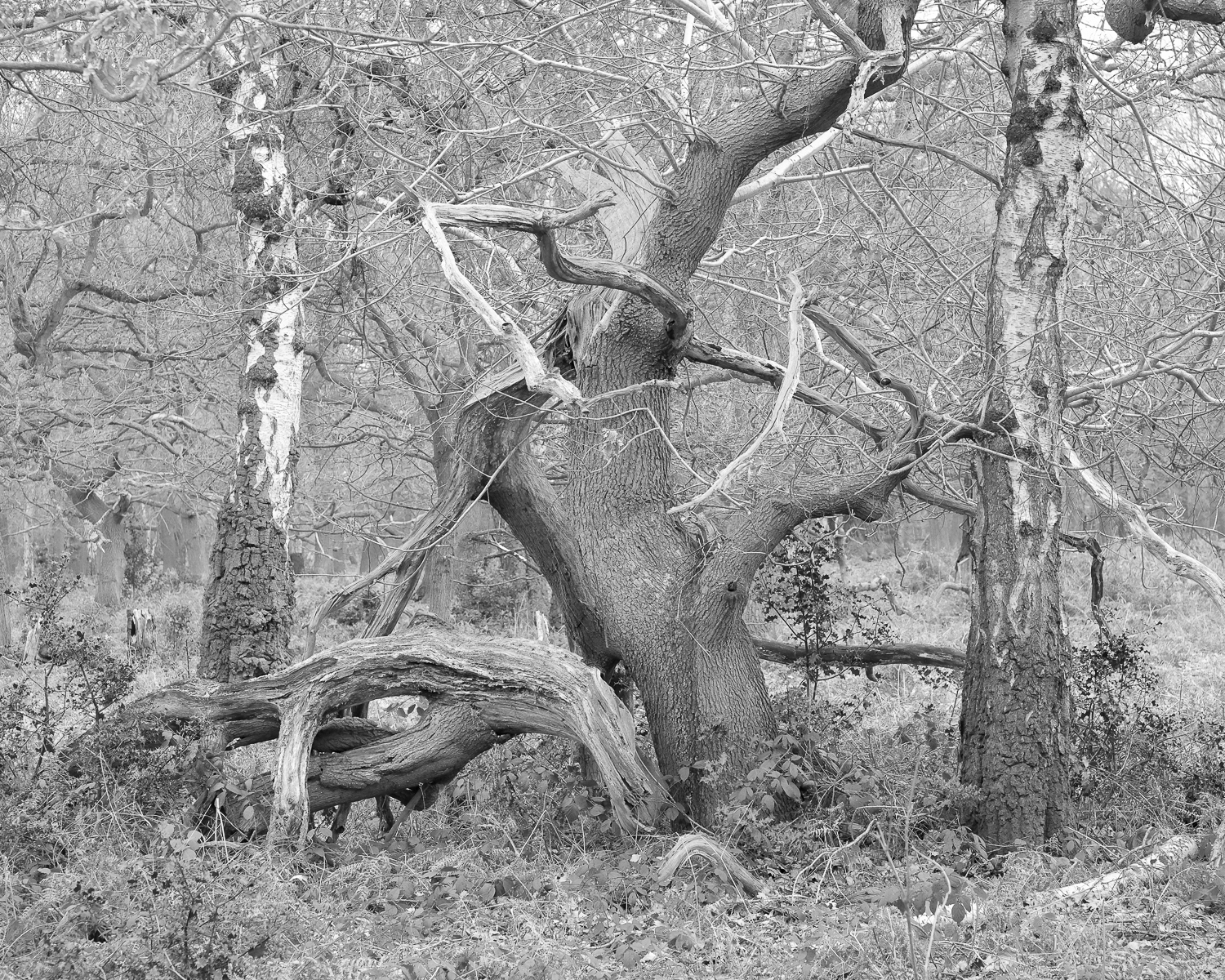Oak and Silver Birches.  The Birklands.

Sciryuda.  Sherwood Forest, Nottinghamshire.

Sciryuda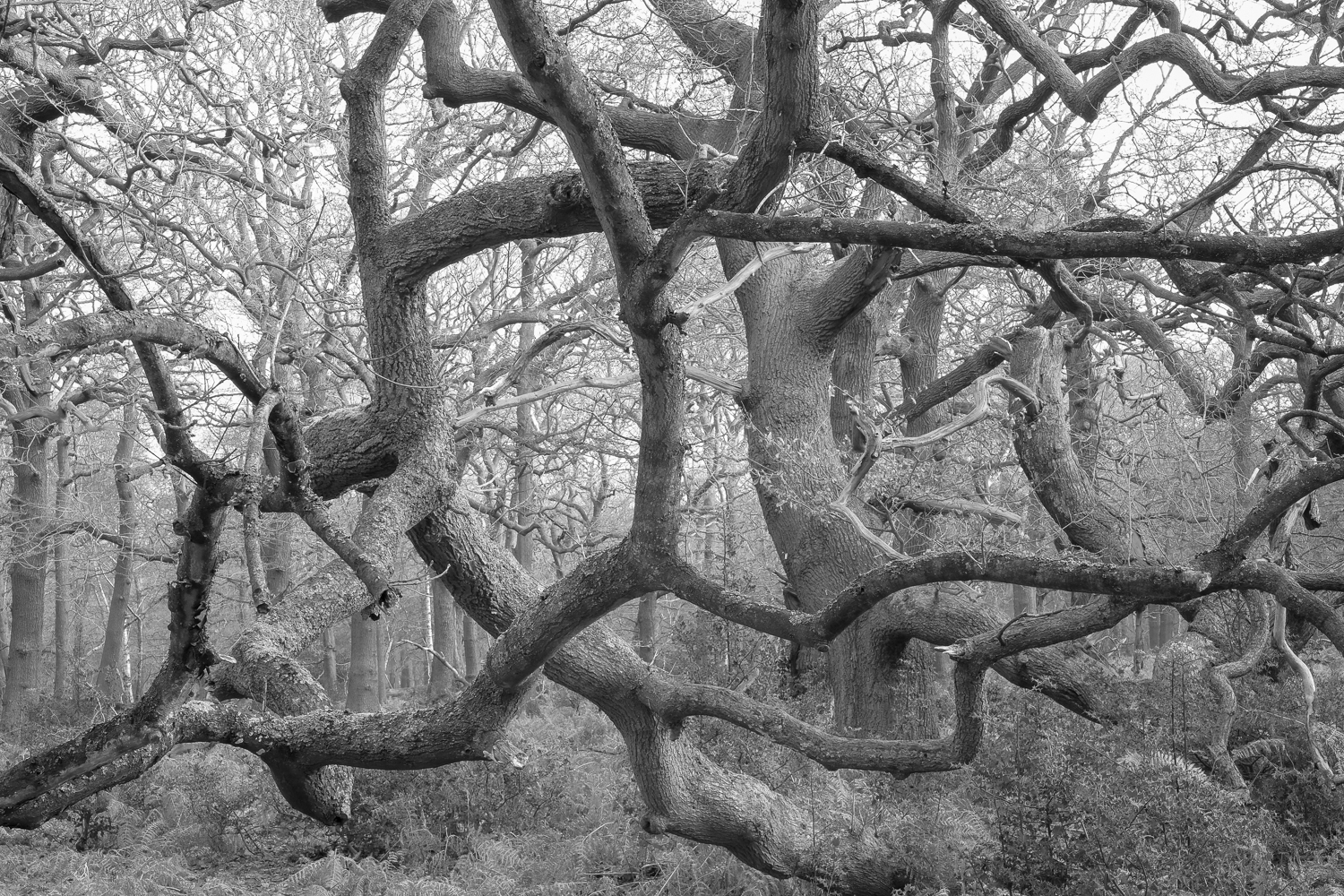Oak.  The Birklands.

Sciryuda, Sherwood Forest, Nottinghamshire.

Sciryuda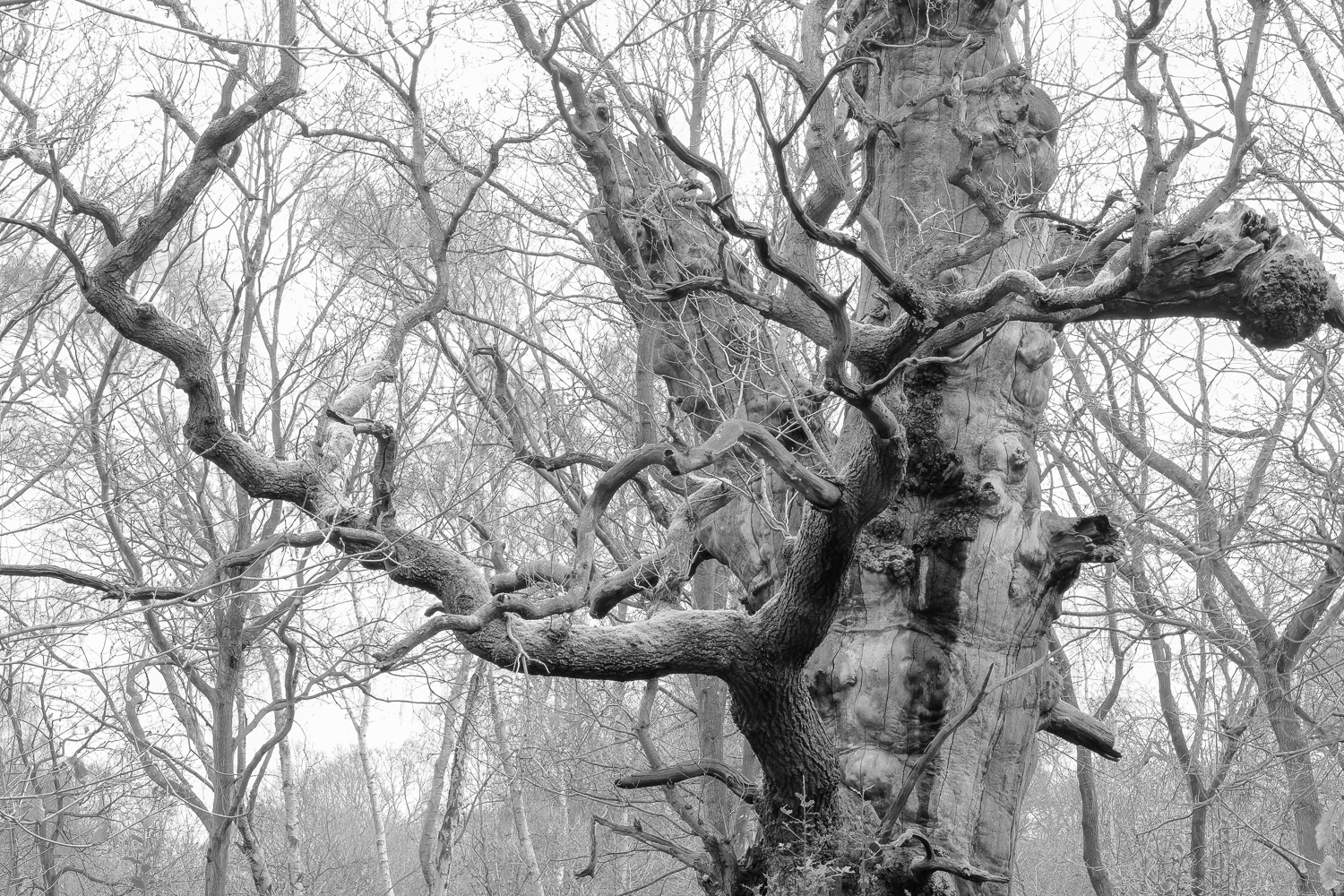Ancient Oak, The Birklands.

Sciryuda. Sherwood Forest, Nottinghamshire.

SciryudaF a l l e n   O a k,   H a n g a r   H i l l   W o o d,   B i r k l a n d s.

S c i r y u d a.   S h e r w o o d   F o r e s t,   N o t t i n g h a m s h i r e.

Sciryuda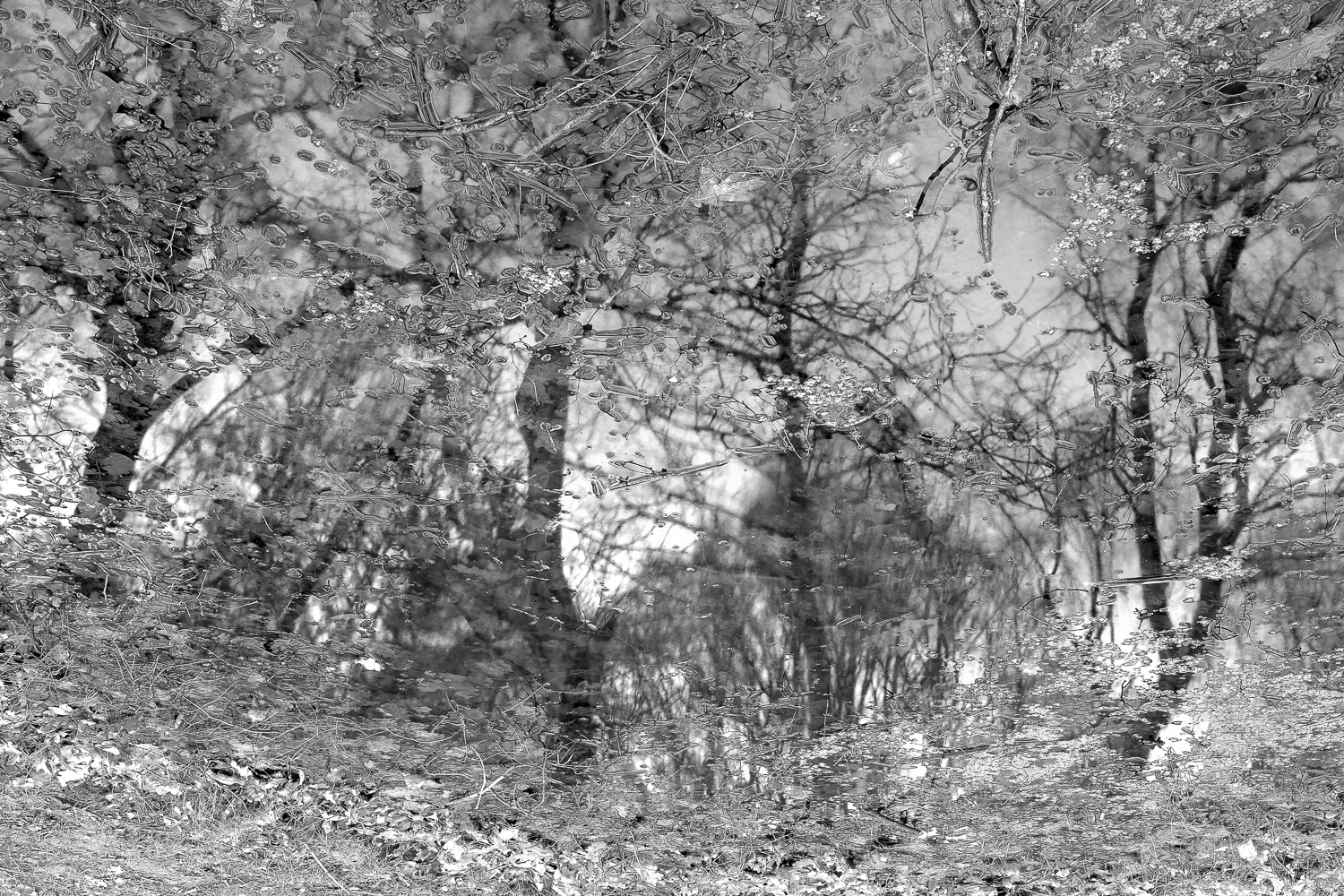P o n d.   C r o w n   H i l l   P l a n t a t i o n,   B u d b y   S o u t h   F o r e s t.

S c i r y u d a.   S h e r w o o d   F o r e s t,   N o t t i n g h a m s h i r e.

Sciryuda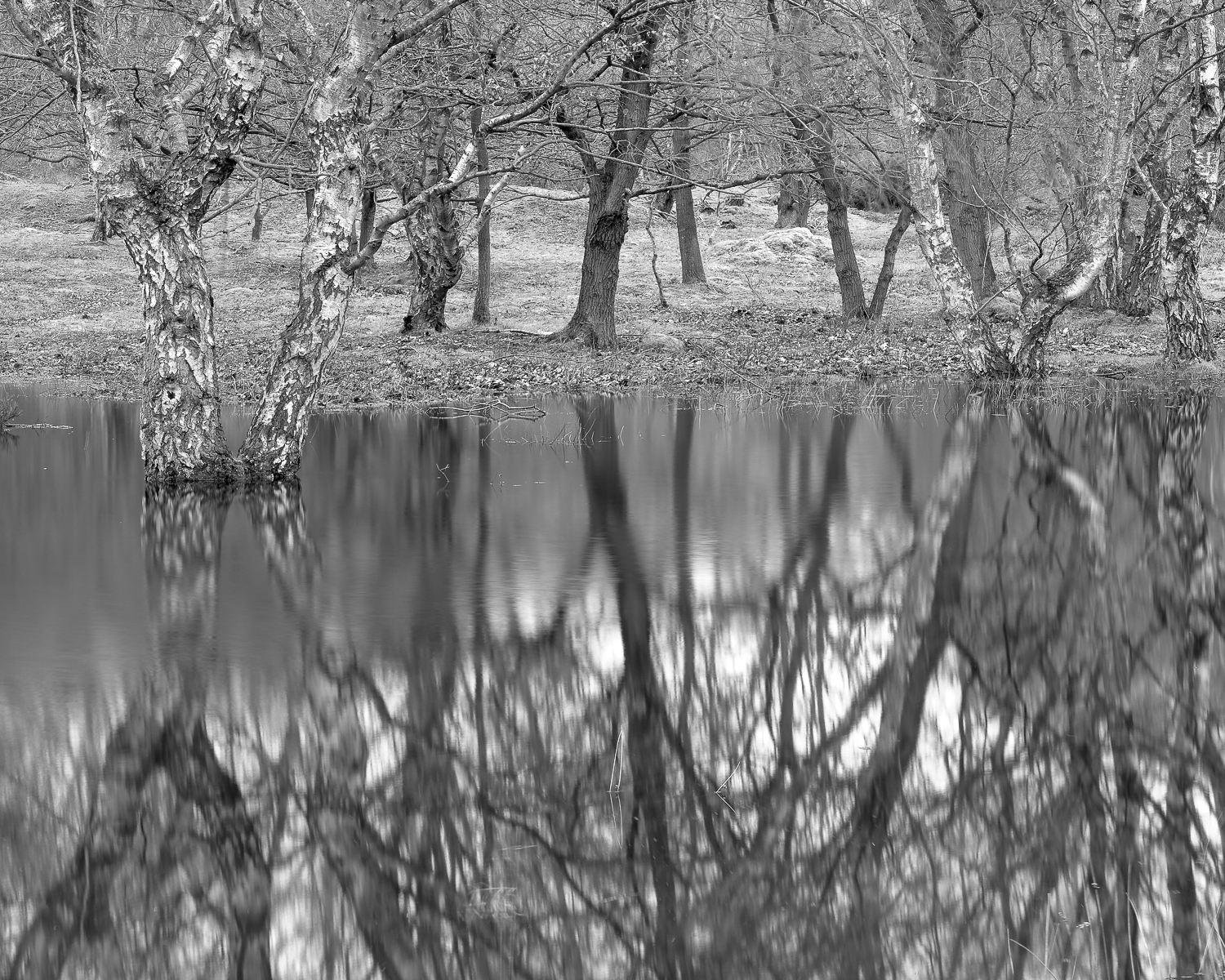S i l v e r   B i r c h   -   N e a r   P i g e o n   P o n d   P l a n t a t i o n.

S c i r y u d a.   S h e r w o o d   F o r e s t,   N o t t i n g h a m s h i r e.

SciryudaO a k.   N e a r   P i g e o n   P o n d   P l a n t a t i o n.

S c i r y u d a.   S h e r w o o d   F o r e s t,   N o t t i n g h a m s h i r e.

Sciryuda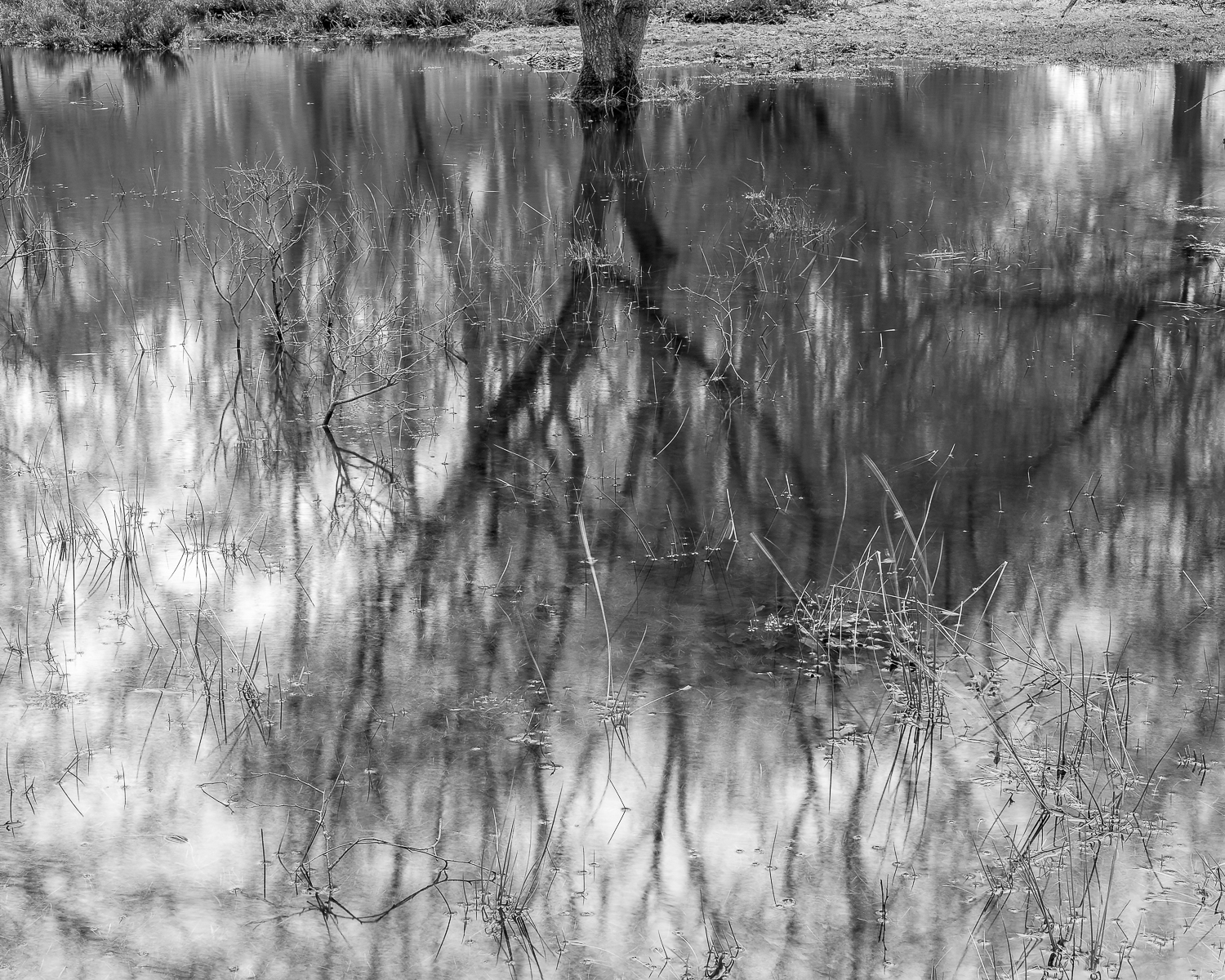O a k.   N e a r   P i g e o n   P o n d   P l a n t a t i o n.

S c i r y u d a,   S h e r w o o d   F o r e s t,   N o t   t   i n g h a m s h i r e.

Sciryuda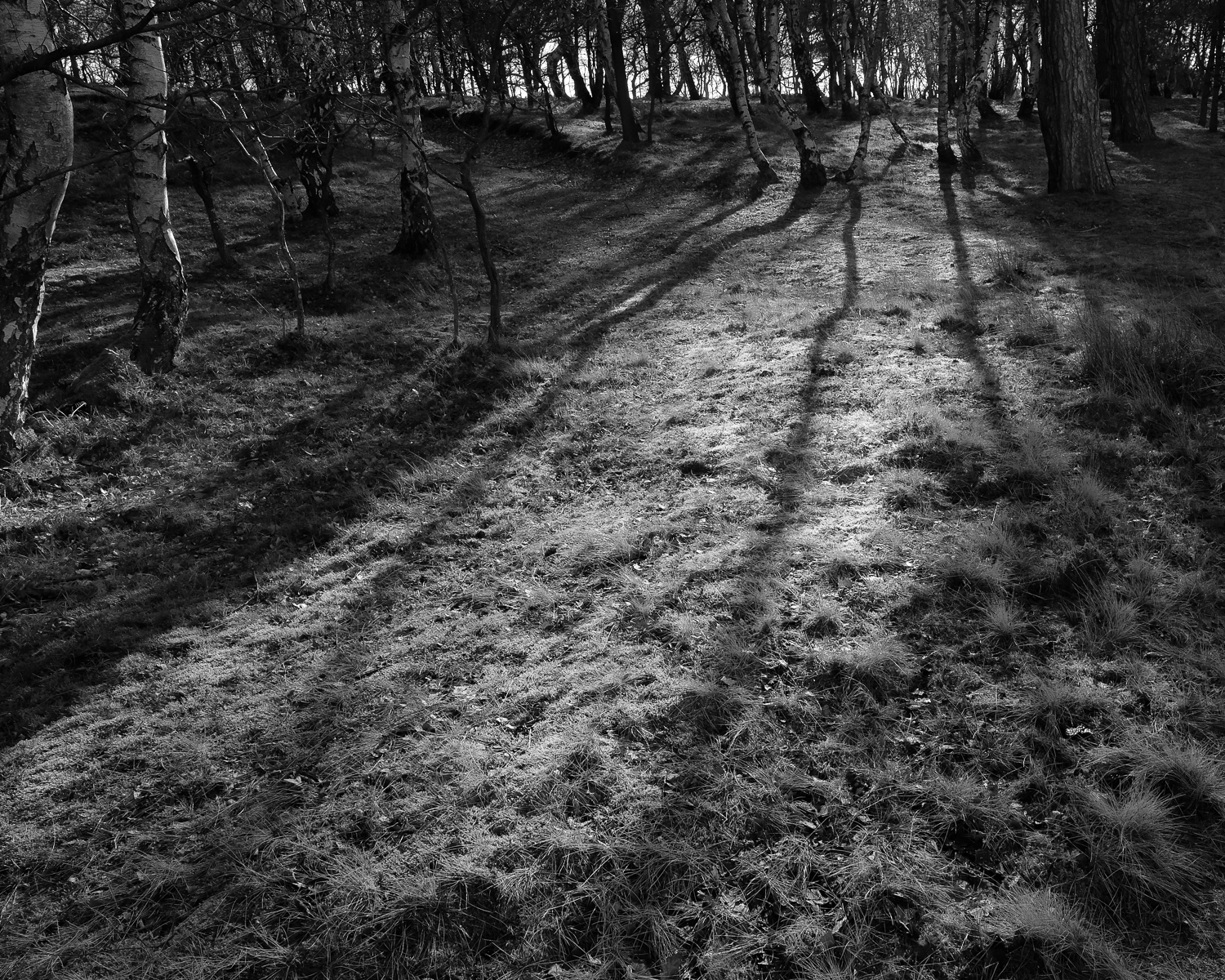C r o w n   H i l l   P l a n t a t i o n,   B u d b y   S o u t h   F o r e s t.

S c i r y u d a,   S h e r w o o d   F o r e s t,   N o t t i n g h a m s h i r e.

Sciryuda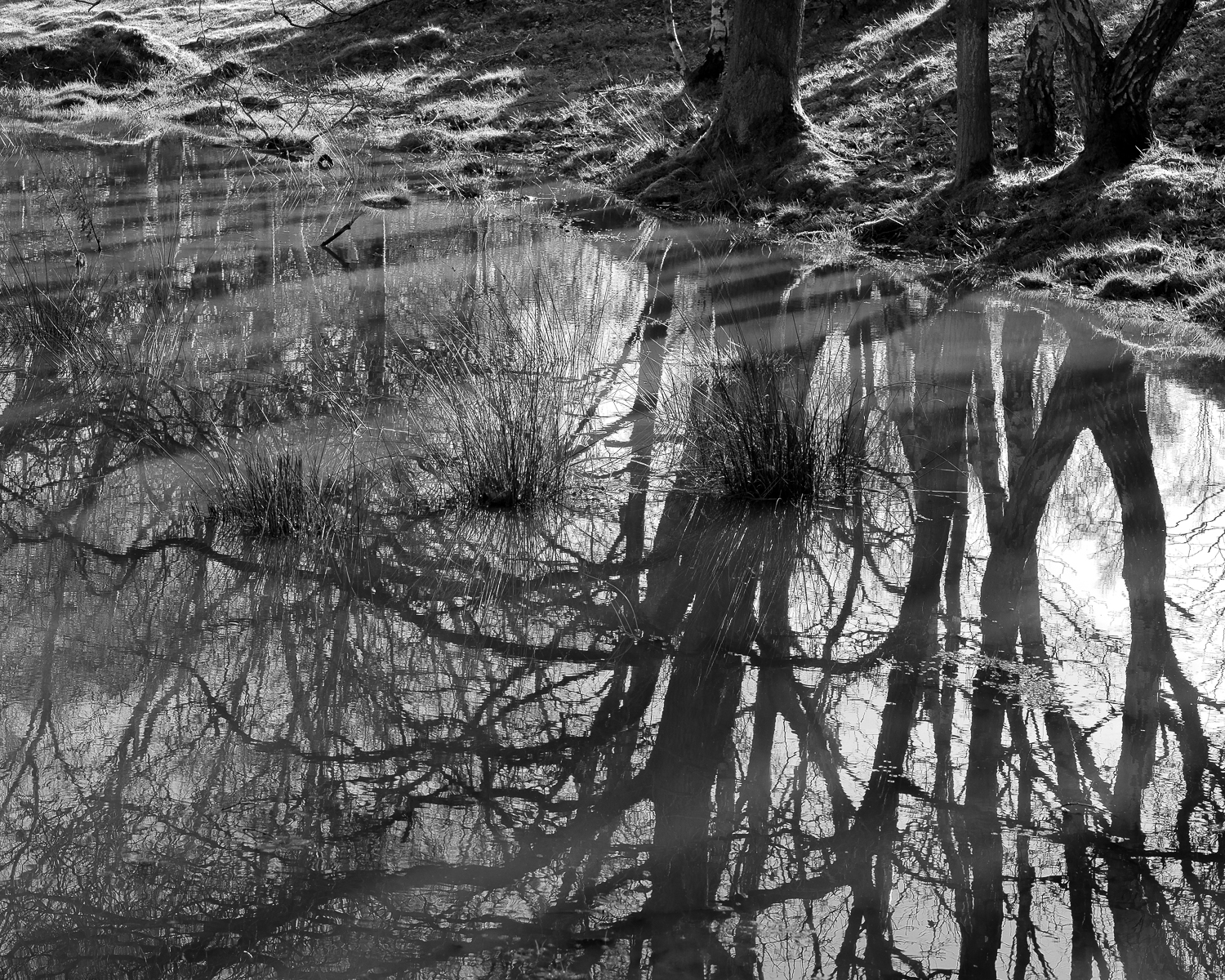B u d b y   S o u t h   F o r e s t

S c i r y u d a.   S h e r w o o d   F o r e s t,   N o t t i n g h a m s h i r e.

Sciryuda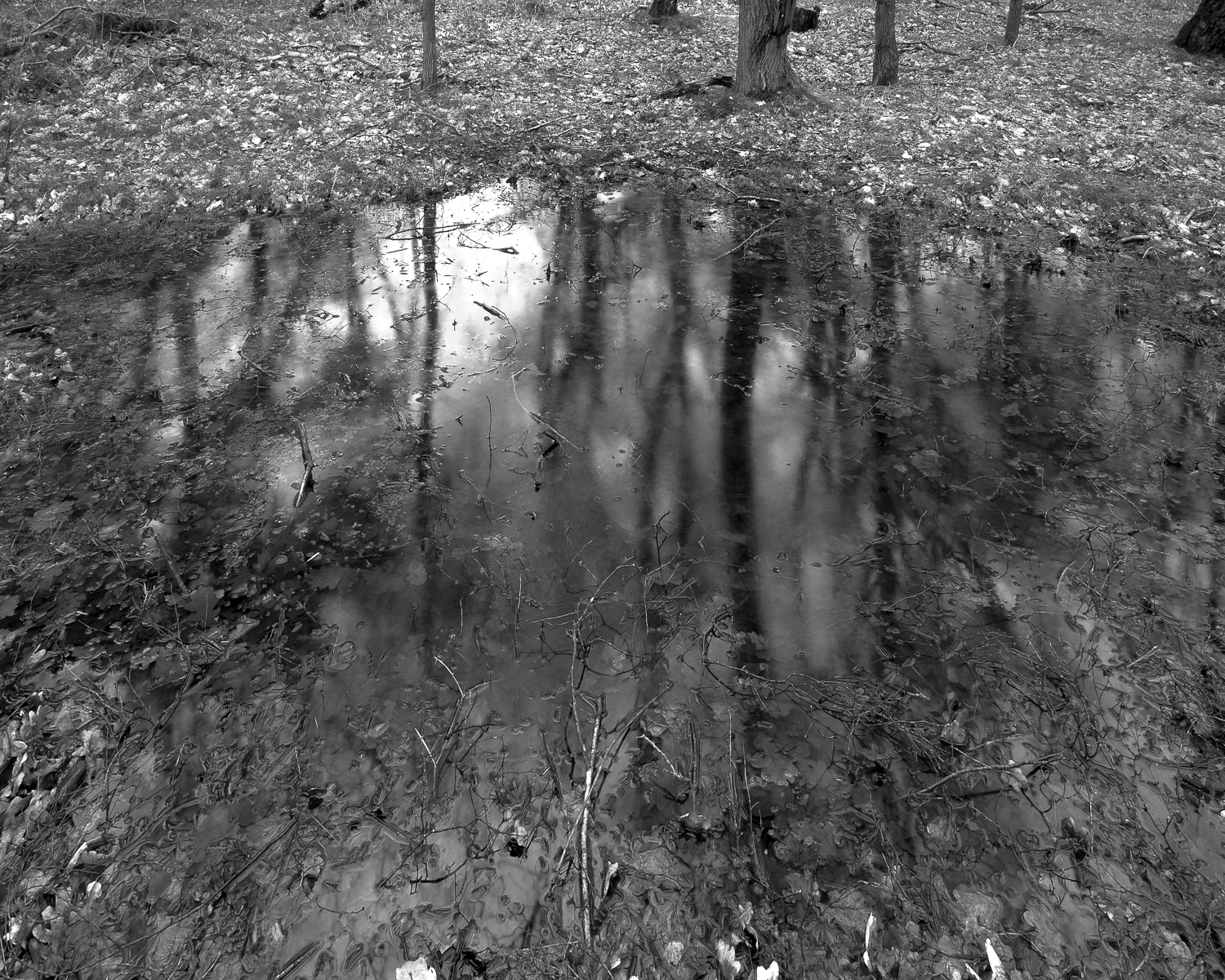P o n d.   C r o w n   H i l l   P l a n t a t i o n.   B u d b y   S o u t h   F o r e s t.

S c i r y u d a,   S h e r w o o d   F o r e s t,   N o t t i n g h a m s h i r e.

Sciryuda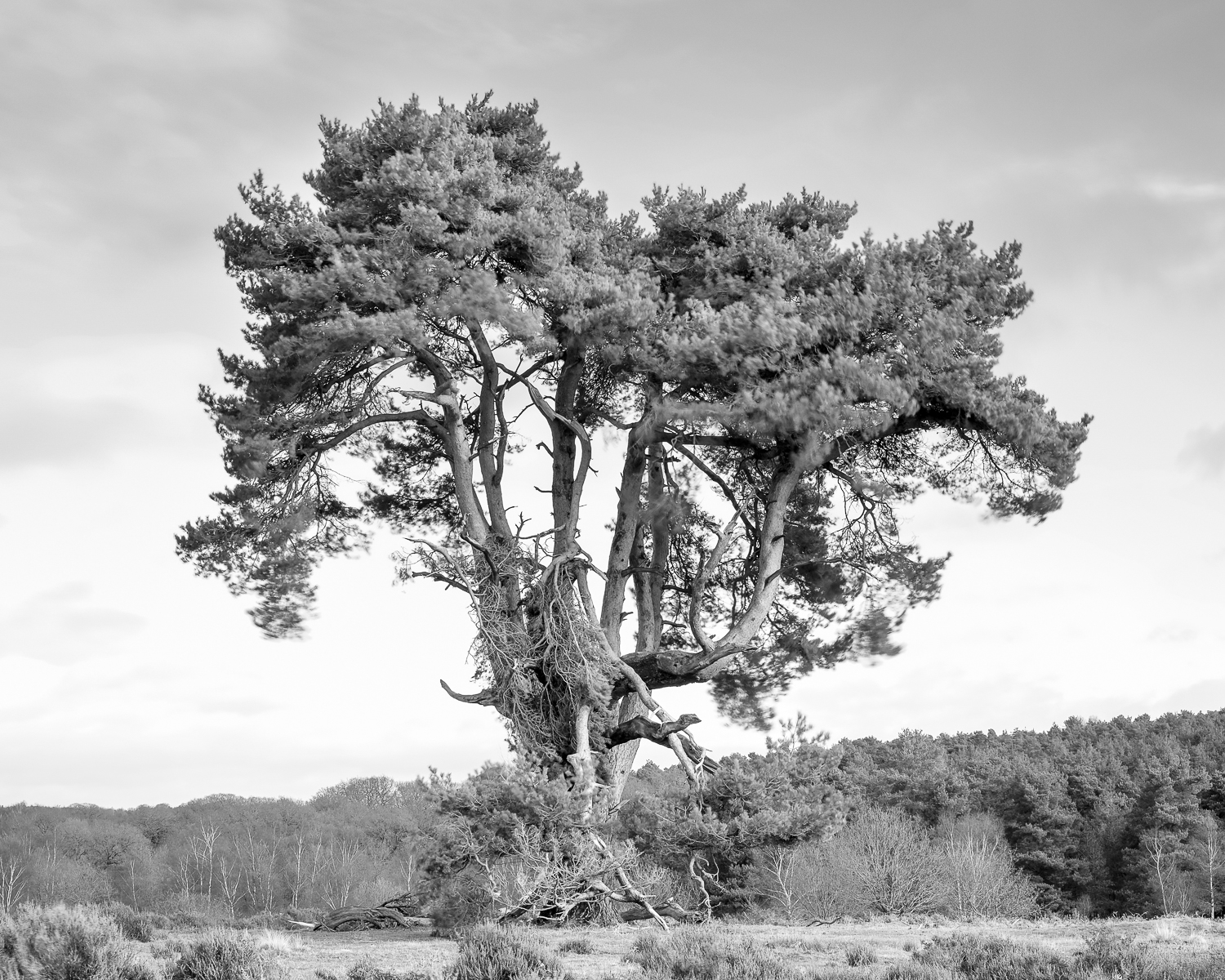S c o t s   P i n e.   B u d b y   S o u t h   F o r e s t.

S c i r y u d a,   S h e r w o o d   F o r e s t,   N o t t i n g h a m s h i r e.

Sciryuda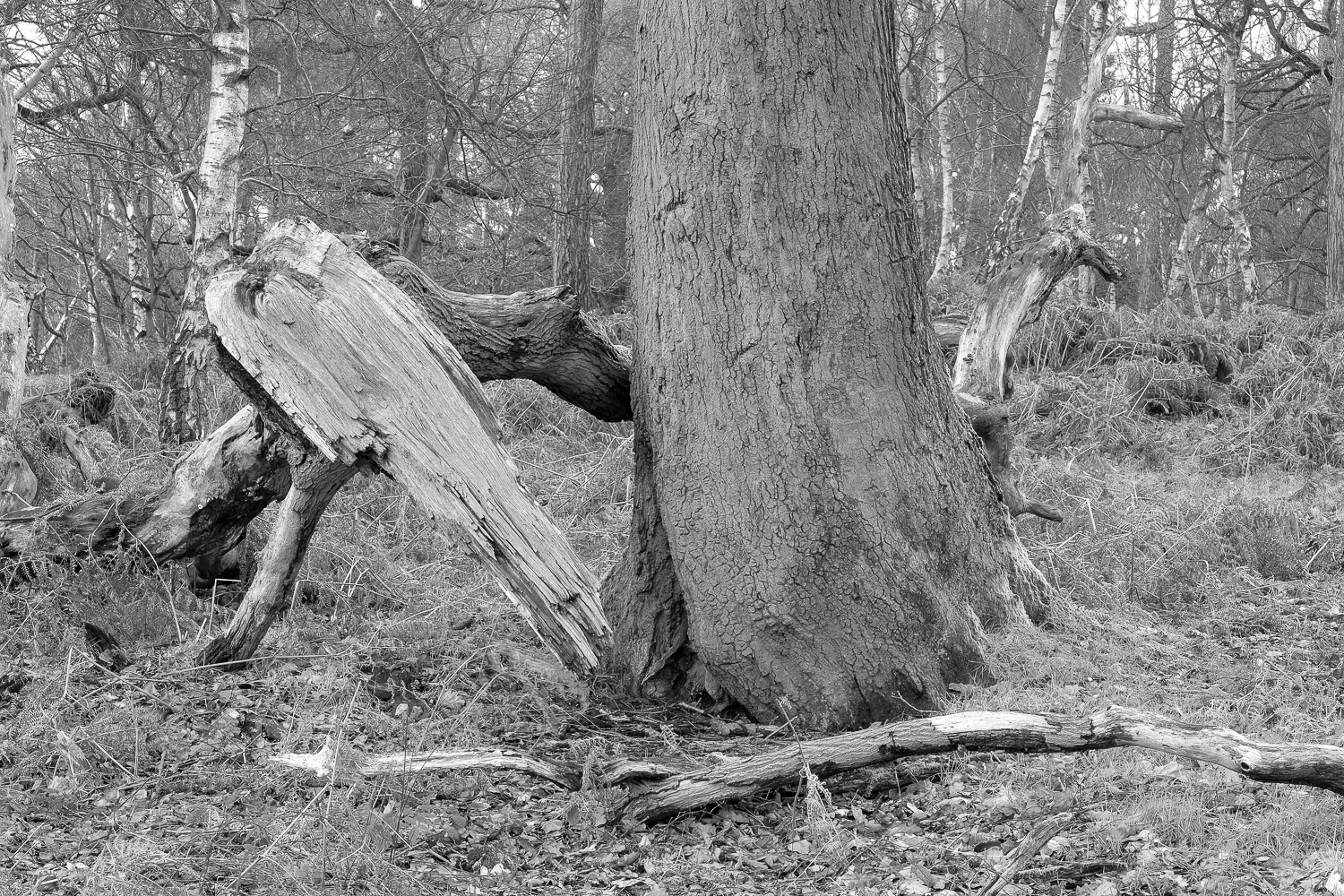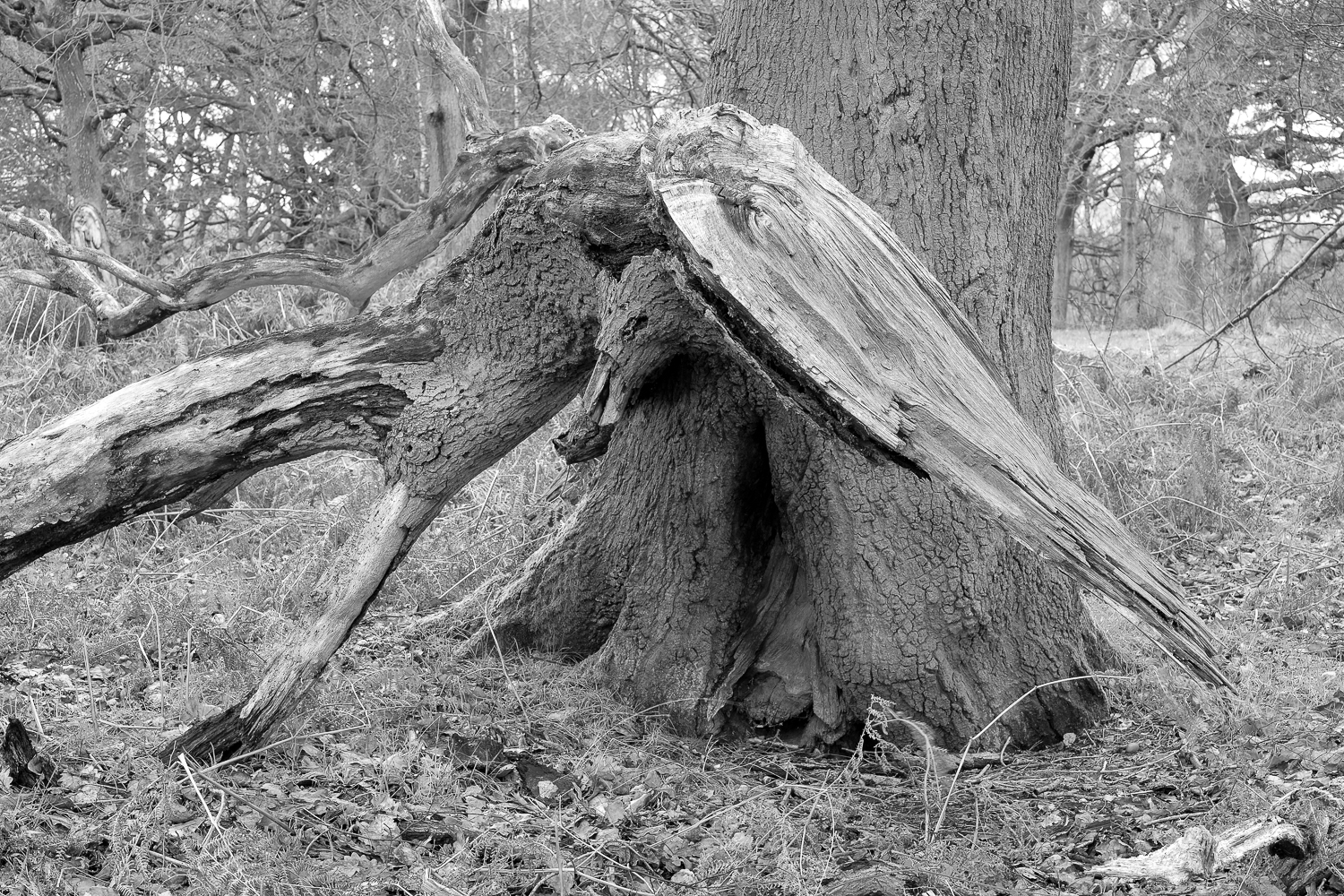S e a r c h i n g.   O a k.   C r o w n   H i l l   P l a n t a t i o n.

S c i r y u d a.   S h e r w o o d   F o r e s t,   N o t t i n g h a m s h i r e.

SciryudaS t o r m.   B u d b y   S o u t h   F o r e s t.

S c i r y u d a.   S h e r w o o d   F o r e s t,   N o t t i n g h a m s h i r e.

Sciryuda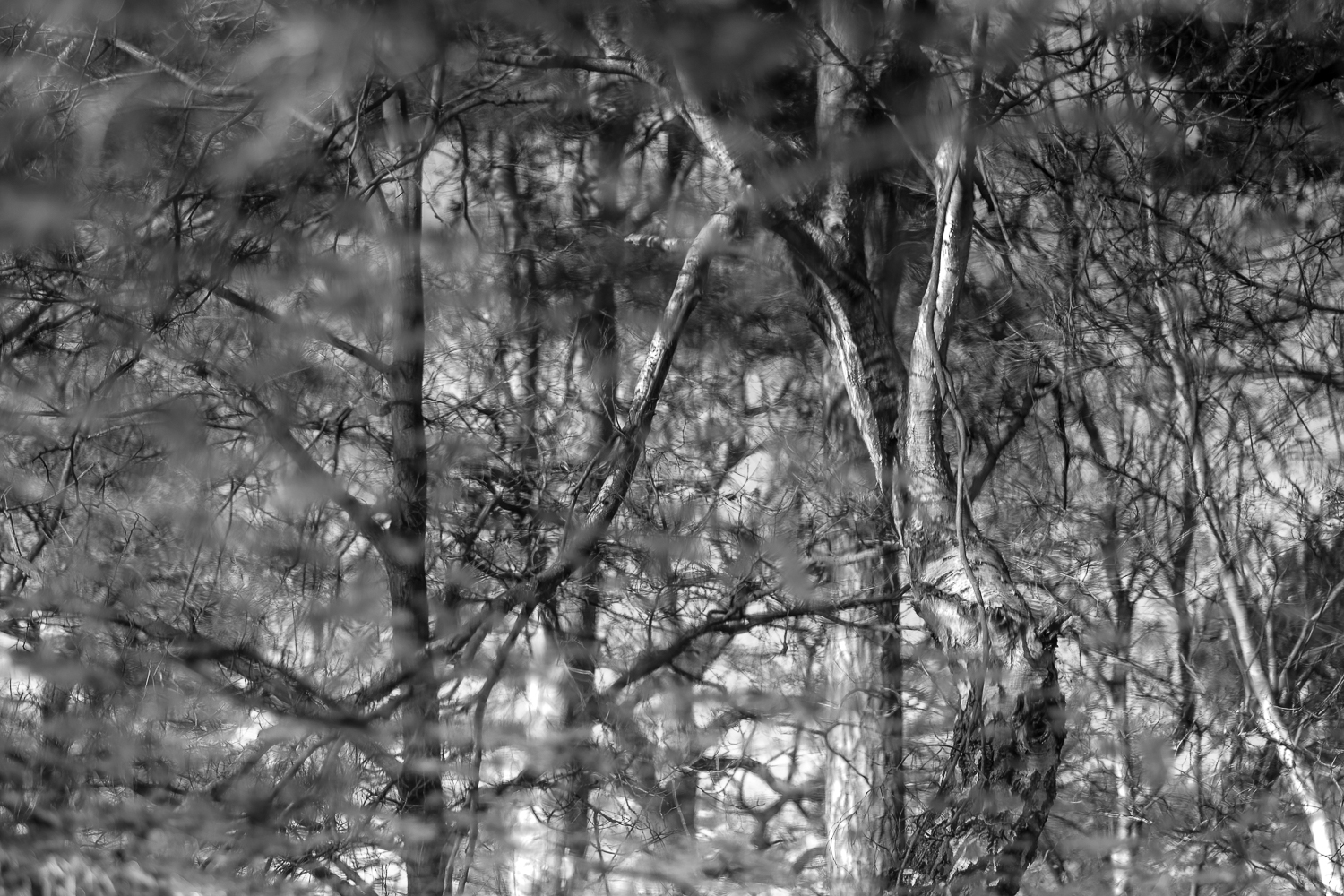B i r c h,   O a k,   P i n e.  W i l d   P o n d.

C r o w n   H i l l   P l a n t a t i o n,   B u d b y   S o u t h   F o r e s t

S c i r y u d a,   S h e r w o o d   F o r e s t,   N o t t i n g h a m s h i r e.

Sciryuda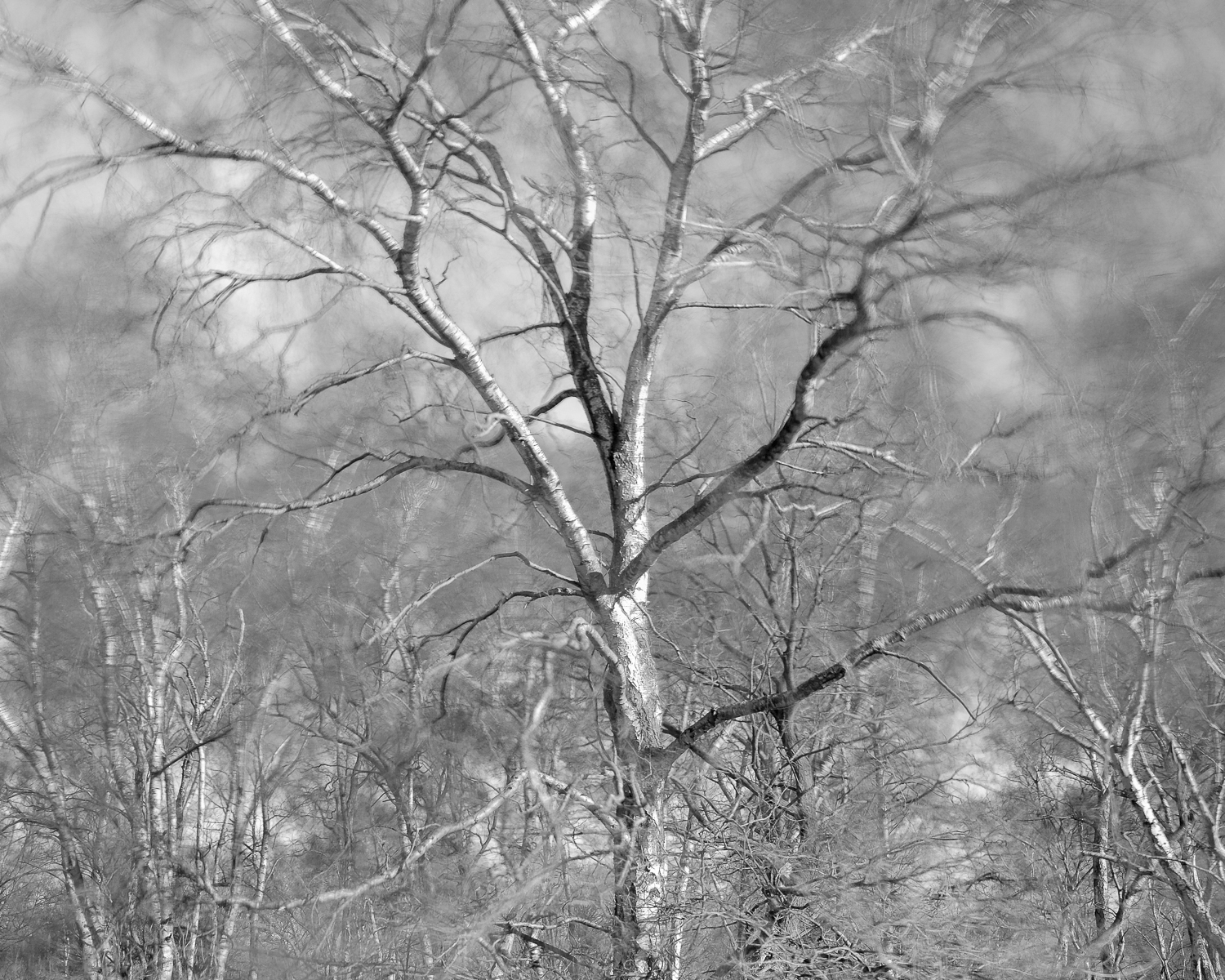S i l v e r   B i r c h   -   ( S t o r m   C i a r a ).   B u r s t h e a r t   H i l l.

S c i r y u d a.   S h e r w o o d   F o r e s t,   N o t t i n g h a m s h i r e.